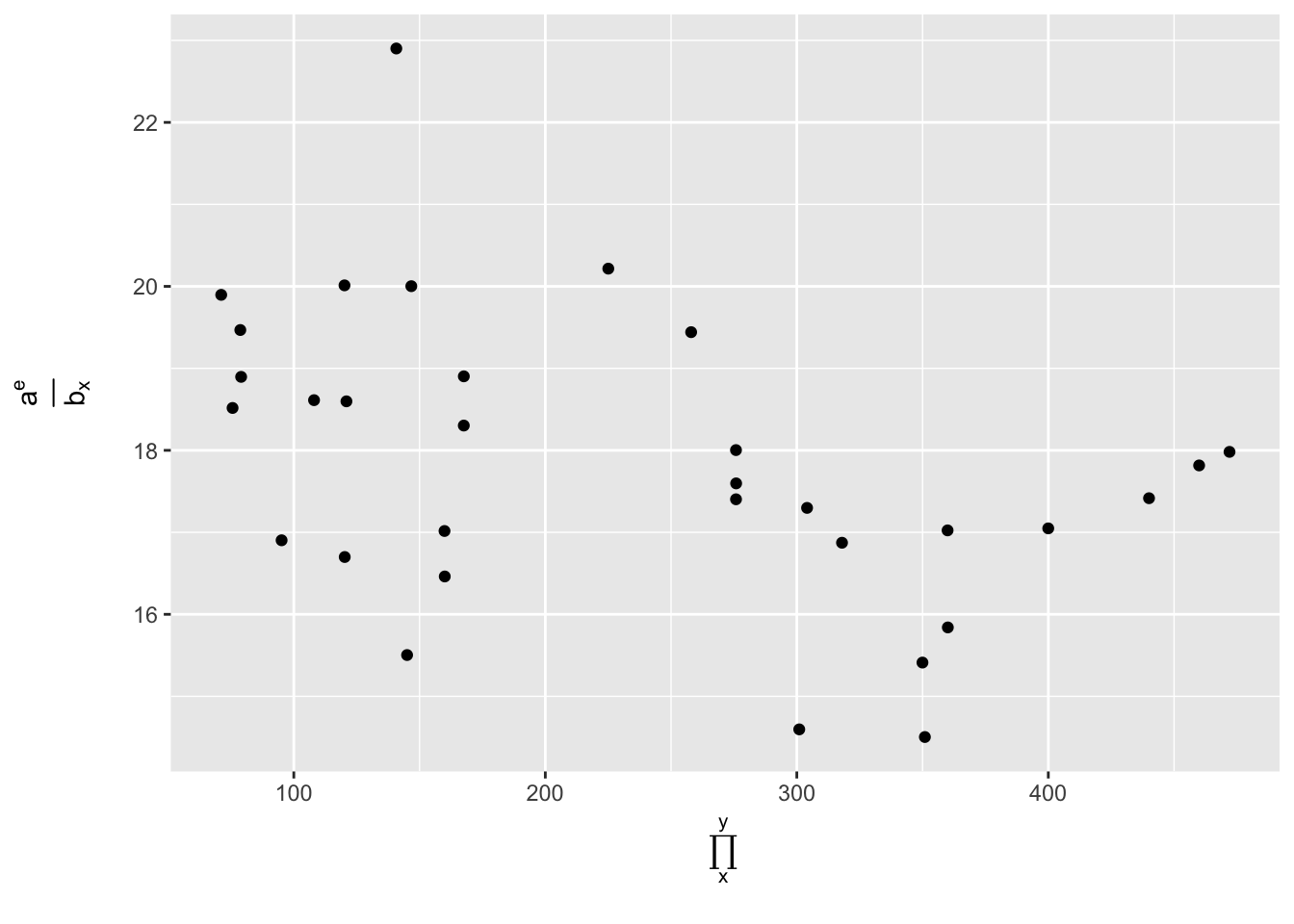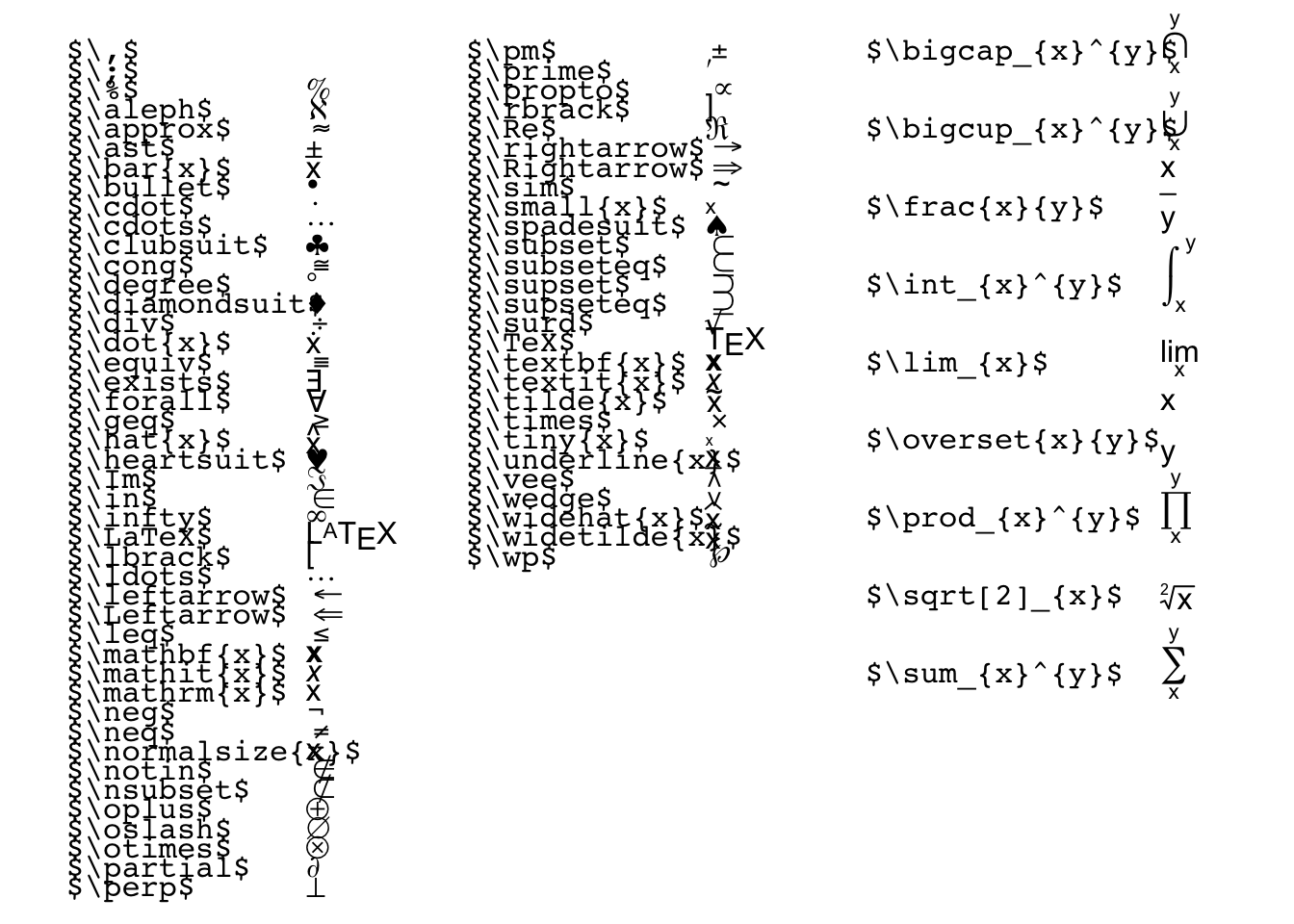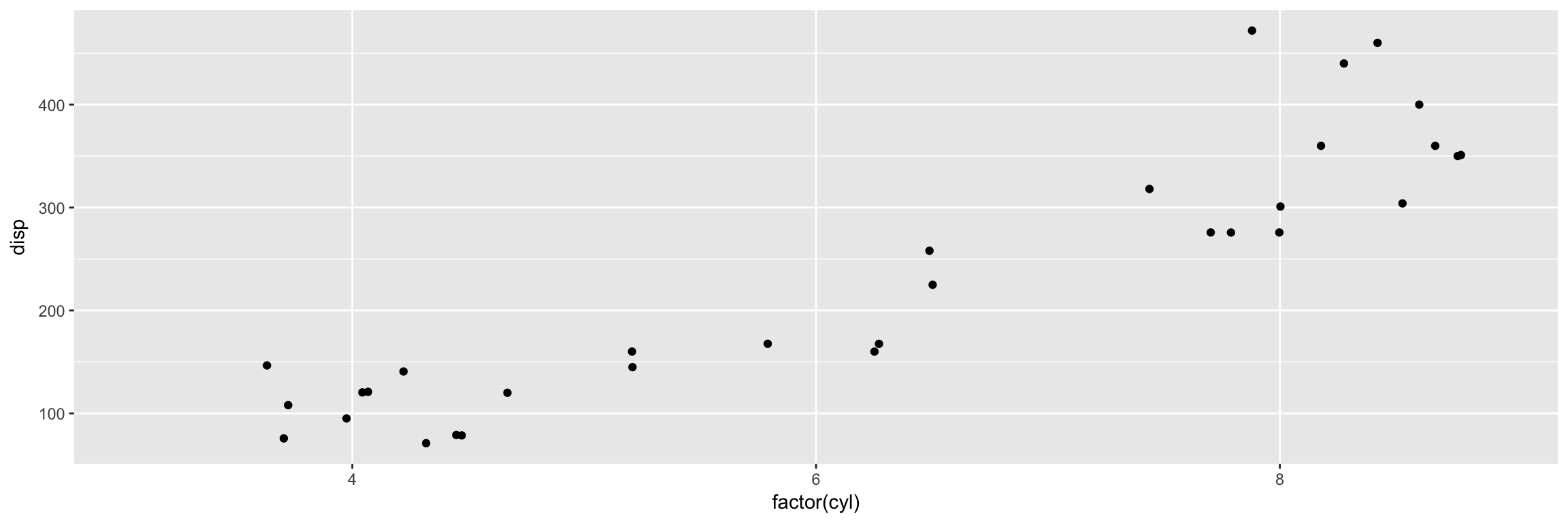# How to publish RMarkdown to WordPress

Create your markdown document in R, using $$\LaTeX$$, knit to html, then copy the file content into WordPress and add at the top the following javascript code:

<script type="text/javascript"
src="http://cdn.mathjax.org/mathjax/latest/MathJax.js?config=TeX-AMS-MML_HTMLorMML">
</script>

This will load images into the blog post itelf and preserve the $$\LaTeX$$ formatting. Simple!
Source

Use the latex2exppackage to translate expression to $$\LaTeX$$ form, that can be added to plots, eg. axes labels or equations in notes.

library (latex2exp)
library(ggplot2)
ggplot(mtcars) + geom_jitter(aes(x=disp, y=qsec)) + ylab(TeX("\\frac{a^e}{b_x}")) + xlab(TeX("\\prod_{x}^{y}"))latex2exp_supported(plot = T)What does NOT work:

Have tried direct export but it does not work. It uses RWordpress, and logs in directly. Still needs the above javascript to preserve $$\LaTeX$$ formatting, and needs additional gymnastics with the plot images. Some additional discussion here.

## Math

Test the Math equation rendering: Inline formula with $$a=3$$ and $$b=5$$ $x = a + b$

## Table

knitr::kable(data.frame(a=1:5,b=rnorm(5)))
a b
1 -0.0499999
2 -1.6248843
3 -0.9676244
4 0.9533118
5 0.6553010
?kable

## Plot

The following plot shows the annual blog frequencies and serves as example of a succesful file upload.

library(ggplot2)
ggplot(mtcars, aes(x=factor(cyl), y=disp)) + geom_jitter()Engine displacement vs cyl count